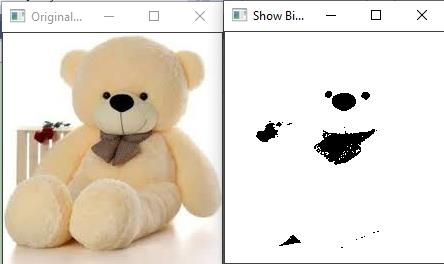# How to create a binary image in OpenCV using C++?

A binary image is just a digital image that represents two colors, black and white. From an image processing perspective, a binary image contains pixels with two possible values- zero and one. When the value of a pixel is 0, it represents a pure black color. When the value of the pixel is 1, it means pure white color.

In a grayscale image, there are 256 different possible values for each. But in Binary Image, there are only two possible values. Binary images have different types of applications. For example, morphological transformation requires a binary image, object shape extraction from background requires a binary image, etc. Using OpenCV, we can freely convert an image into a binary image.

The following example is converting image loaded in 'original_image' matrix into a grayscale image and storing it into 'grayscale_image' matrix-

cvtColor(original_image, grayscale_image, COLOR_BGR2GRAY);

The next line is converting the grayscale image into a binary image and storing the converted image into 'binary_image' matrix.

threshold(grayscale_image, binary_image, 100, 255, THRESH_BINARY);

Here 'grayscale_image' is the source matrix, 'binary_image' is the destination matrix. After that, there are two values 100 and 255. These two values represent the threshold range. In this line, the threshold range represents the grayscale pixel values to be converted.

The following program loads an image and converts it into a binary image.

## Example

#include<iostream>
#include<opencv2/highgui/highgui.hpp>
#include<opencv2/imgproc/imgproc.hpp>
using namespace cv;
using namespace std;
int main(int argc, char** argv) {
Mat original_image;//declaring a matrix to load the original image//
Mat grayscale_image;//declaring a matrix to store grayscale image//
Mat binary_image;//declaring a matrix to store the binary image
namedWindow("Original Image");//declaring window to show binary image//
namedWindow("Show Binary");//declaring window to show original image//
cvtColor(original_image, grayscale_image, COLOR_BGR2GRAY);//Converting BGR to Grayscale image and storing it into converted matrix//
threshold(grayscale_image, binary_image, 100, 255, THRESH_BINARY);//converting grayscale image stored in converted matrix into binary image//
imshow("Original Image", original_image);//showing Original Image//
imshow("Show Binary", binary_image);//showing Binary Image//
waitKey(0);
return 0;
}

## Output